Publicité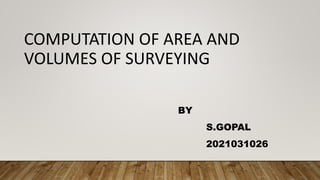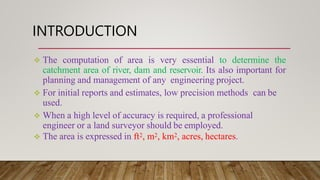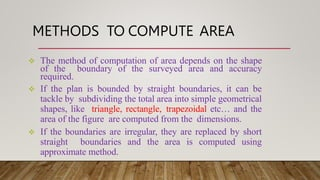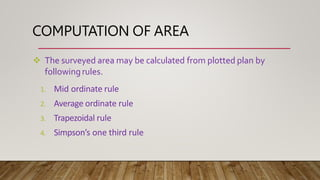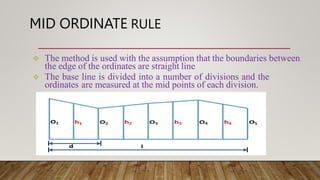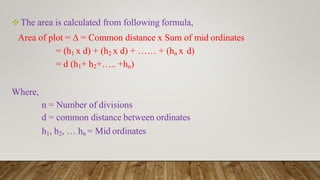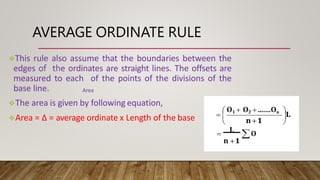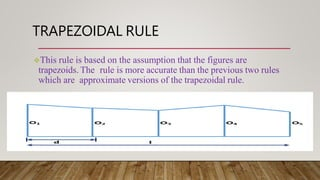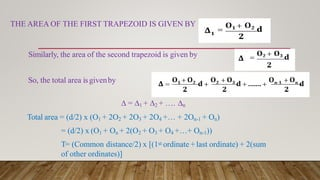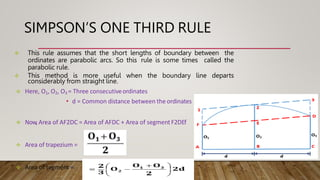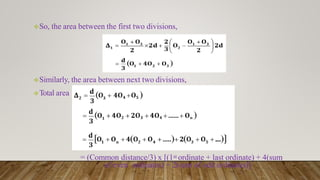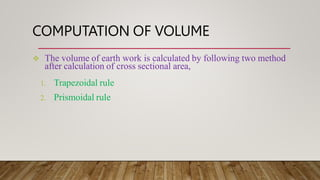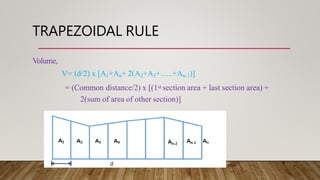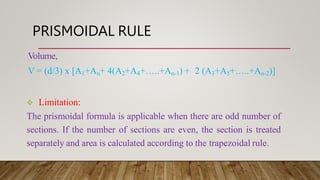1 sur 15
Publicité

### 2021031026 S GOPAL SWE.pptx

1. COMPUTATION OF AREA AND VOLUMES OF SURVEYING BY S.GOPAL 2021031026
2. INTRODUCTION  The computation of area is very essential to determine the catchment area of river, dam and reservoir. Its also important for planning and management of any engineering project.  For initial reports and estimates, low precision methods can be used.  When a high level of accuracy is required, a professional engineer or a land surveyor should be employed.  The area is expressed in ft2, m2, km2, acres, hectares.
3. METHODS TO COMPUTE AREA  The method of computation of area depends on the shape of the boundary of the surveyed area and accuracy required.  If the plan is bounded by straight boundaries, it can be tackle by subdividing the total area into simple geometrical shapes, like triangle, rectangle, trapezoidal etc… and the area of the figure are computed from the dimensions.  If the boundaries are irregular, they are replaced by short straight boundaries and the area is computed using approximate method.
4. COMPUTATION OF AREA  The surveyed area may be calculated from plotted plan by followingrules. 1. Mid ordinate rule 2. Average ordinate rule 3. Trapezoidal rule 4. Simpson’s one third rule
5. MID ORDINATE RULE  The method is used with the assumption that the boundaries between the edge of the ordinates are straight line  The base line is divided into a number of divisions and the ordinates are measured at the mid points of each division.
6. The area is calculated from following formula, Area of plot = ∆ = Common distance x Sum of mid ordinates = (h1 x d) + (h2 x d) + …… + (hn x d) = d (h1+ h2+….. +hn) Where, n = Number of divisions d = common distance between ordinates h1, h2, … hn = Mid ordinates
7. AVERAGE ORDINATE RULE This rule also assume that the boundaries between the edges of the ordinates are straight lines. The offsets are measured to each of the points of the divisions of the base line. The area is given by following equation, Area = ∆ = average ordinate x Length of the base Area
8. TRAPEZOIDAL RULE This rule is based on the assumption that the figures are trapezoids. The rule is more accurate than the previous two rules which are approximate versions of the trapezoidal rule.
9. THE AREA OF THE FIRST TRAPEZOID IS GIVEN BY Similarly, the area of the second trapezoid is given by So, the total area isgivenby ∆ = ∆1 + ∆2 + …. ∆n Total area = (d/2) x (O1 + 2O2 + 2O3 + 2O4 +… + 2On-1 + On) = (d/2) x (O1 + On + 2(O2 + O3 + O4 +…+ On-1)) T= (Common distance/2) x [(1st ordinate +last ordinate) + 2(sum of other ordinates)]
10. SIMPSON’S ONE THIRD RULE  This rule assumes that the short lengths of boundary between the ordinates are parabolic arcs. So this rule is some times called the parabolic rule.  This method is more useful when the boundary line departs considerably from straight line.  Here, O1, O2, O3 = Three consecutiveordinates • d = Common distance between the ordinates  Now , Area of AF2DC = Area of AFDC + Area of segmentF2DEf  Area of trapezium =  Area of segment =
11. So, the area between the first two divisions, Similarly, the area between next two divisions, Total area = (Common distance/3) x [(1st ordinate + last ordinate) + 4(sum of even ordinates) + 2(sum of odd ordinates)]
12. COMPUTATION OF VOLUME  The volume of earth work is calculated by following two method after calculation of cross sectional area, 1. Trapezoidal rule 2. Prismoidal rule
13. TRAPEZOIDAL RULE Volume, V= (d/2) x [A1+An+ 2(A2+A3+…..+An-1)] = (Common distance/2) x [(1st section area + last section area) + 2(sum of area of other section)] d
14. PRISMOIDAL RULE Volume, V = (d/3) x [A1+An+ 4(A2+A4+…..+An-1) + 2 (A3+A5+…..+An-2)]  Limitation: The prismoidal formula is applicable when there are odd number of sections. If the number of sections are even, the section is treated separately and area is calculated according to the trapezoidal rule.
15. THANK YOU
Publicité